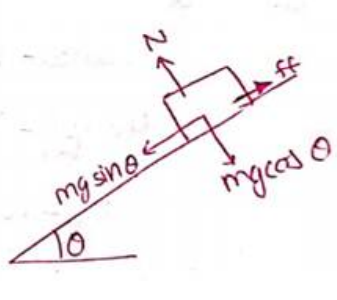Deepak Scored 45->99%ile with Bounce Back Crack Course. You can do it too!

# Solve the following :

Question:

The friction coefficient between a road and the type of a vehicle is $4 / 3$. Find the maximum incline the road may have so that once hard brakes are applied and the wheel starts skidding, the vehicle going down at a speed of $36 \mathrm{~km} / \mathrm{hr}$ is stopped within $5 \mathrm{~m}$.

Solution:$u=36 \times \frac{5}{18}=10 \mathrm{~m} / \mathrm{s}$

$v=0 ; s=5 \mathrm{~m}$

$v^{2}=u^{2}+2 a s$

$0^{2}=(10)^{2}+2(a)(5)$

$a=-10 \mathrm{~m} / \mathrm{s}^{2}$

Now, by translatory motion equation

$m g \sin \theta-f f=m a$

$m g \sin \theta-\mu N=m a$

$m g \sin \theta-\mu m g \cos \theta=m a$

$10 \sin \theta-\frac{4}{3} \times 10 \times \cos \theta=(-10)$

$\sin \theta+1=\frac{4}{3} \sqrt{1-\sin ^{2} \theta}$

Squaring

$9(1+\sin \theta)^{2}=16\left(1-\sin ^{2} \theta\right)$

$9\left(1+\sin ^{2} \theta+2 \sin \theta\right)=16-16 \sin ^{2} \theta$

$25 \sin ^{2} \theta+18 \sin \theta-7=0$

On solving,

$\theta=16^{\circ}$